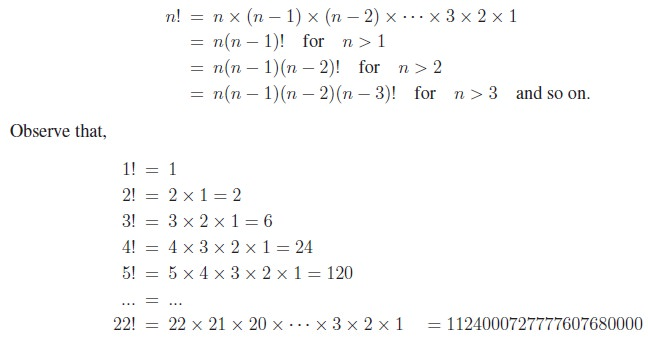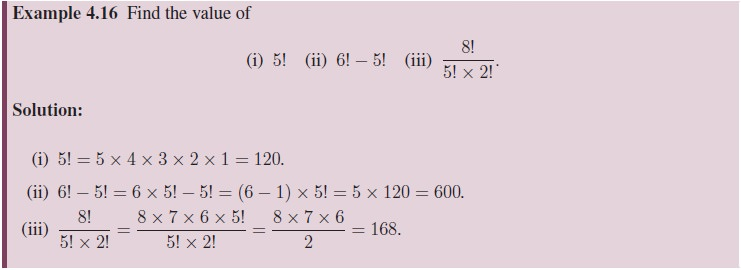Home | | Maths 11th std | Factorials

# Factorials

Factorial of a natural number n is the product of the first n natural numbers.

Factorials

Factorial of a natural number n is the product of the first n natural numbers. It is denoted by n!.

That is,

n!=1 × 2 × 3 ×···× n.

We read this symbol as “n factorial” or “factorial of n”. The notation n! was introduced by the French mathematician Christian Kramp in the year 1808. Note that for a positive integer nThe number 22 ( the Birth date of Ramanujan) has a special place with respect to factorial that, it is the least integer N greater than 1 whose factorial has exactly N digits.

It will be a good exercise for both students and teachers to find the next number N such that N! has exactly N digits.

Note that 0! = 1 is evident by substituting n = 0 in the equation (n + 1)! = (n + 1) × n! as 1! = (0 + 1) × 0! 0! = 1! / 1 = 1. This way, we talk of factorial for non-negative integers. Note that factorials can be extended to certain negative numbers and also to complex numbers, which are beyond the scope of this book.

We shall now discuss certain examples in order to familiarise the computation of factorials.Tags : Definition, Formula, Solved Example Problems, Exercise | Mathematics , 11th Mathematics : UNIT 4 : Combinatorics and Mathematical Induction
Study Material, Lecturing Notes, Assignment, Reference, Wiki description explanation, brief detail
11th Mathematics : UNIT 4 : Combinatorics and Mathematical Induction : Factorials | Definition, Formula, Solved Example Problems, Exercise | Mathematics